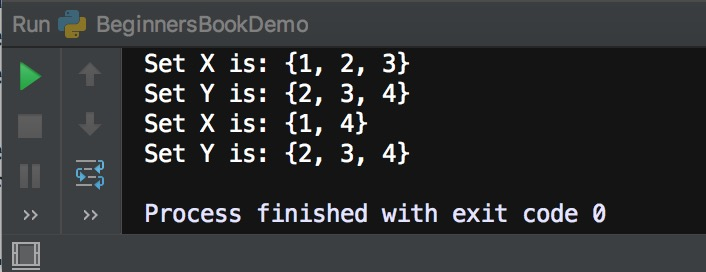In the last tutorial we discussed symmetric_difference() method which returns a new set which is a symmetric difference of two given sets. Here we will discuss, symmetric_difference_update() method which does not return anything but it updates the calling set with the symmetric difference set. For example calling this method like this A.symmetric_difference_update(B) would update set A with the symmetric difference set.

## Set symmetric_difference_update() Syntax

`set.symmetric_difference_update(another_set)`

Parameter: It takes a set as a parameter
Return Value: It does not return anything, it just updates the calling set.

## Python Set symmetric_difference_update() Example

In the following example we have two sets X and Y. We are calling symmetric_difference_update() method like this X.symmetric_difference_update(Y) which finds the symmetric difference between X and Y, which contains the elements that are either in Set X or in Set Y but not in both, this method then updates the calling set X with the symmetric difference.

```# Set X
X = {1, 2, 3}

# Set Y
Y = {2, 3, 4}

# Before calling symmetric_difference_update()
print("Set X is:", X)
print("Set Y is:", Y)

# calling symmetric_difference_update() method
X.symmetric_difference_update(Y)

# After calling symmetric_difference_update()
print("Set X is:", X)
print("Set Y is:", Y)```

Output:Share.